## Example Questions

### Example Question #142 : Fractions

Convertto a mixed number.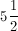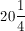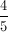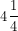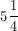Explanation:

4 goes into 21 five times. 5 becomes your whole number. There is a remainder of 1 and your denominator remains the same, so your fraction is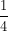.### Example Question #1 : How To Find Out A Mixed Fraction From An Improper Fraction

What is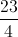written as a mixed number?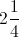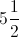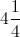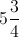Explanation:goes intofive times with a remainder ofThe denominator does not change.

### Example Question #3 : Mixed / Improper Fractions

Which of the following is the mixed fraction equivalent to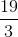?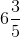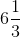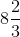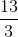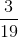Explanation:

To begin, notice that using your calculator, you can find: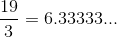Now, the closest even multiple ofthat is less thanis.  Therefore, you know that your number is: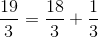This is the same as: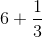, or simply,.  This is your mixed fraction.

### Example Question #1 : How To Find Out A Mixed Fraction From An Improper Fraction

Which of the following is equivalent to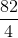?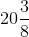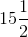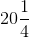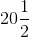Explanation:

Although there are many ways to convert improper fractions into mixed fractions, the easiest way is to use your calculator to your advantage.  Begin by dividing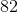by.  This gives you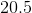. Therefore, you can eliminate all the options that have do not havefor their first portion. Next, multiplyby the denominator (), and get.  This means that you haveand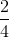, or.  Thus, your answer is.

### Example Question #2 : How To Find Out A Mixed Fraction From An Improper Fraction

What does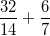equal, expressed as a mixed number?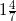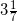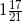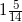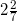Explanation:

Simplify the two fractions, then find a common denominator, then solve.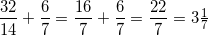### Example Question #3 : How To Find Out A Mixed Fraction From An Improper Fraction

Convert the improper fraction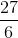to a mixed number. Reduce all fractions if possible.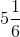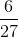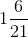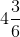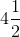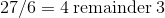.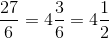when we reduce.